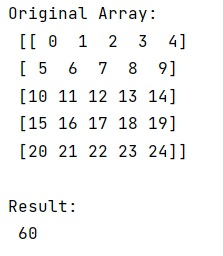# numpy.einsum() Method with Example

Learn about the numpy.einsum() method with its usage, syntax, and example.
Submitted by Pranit Sharma, on March 21, 2023

## numpy.einsum() Method

The numpy.einsum() method is used to evaluate Einstein's summation convention on operands. The Einstein summation convention is a simple fashion to represent many common multi-dimensional, linear algebraic array operations.

The numpy.einsum() computes these values in implicit mode but in explicit mode, einsum provides further flexibility to compute other array operations that might not be considered classical Einstein summation operations, by disabling or forcing summation over specified subscript labels.

## Syntax

```numpy.einsum(subscripts, *operands, out=None, dtype=None,
order='K', casting='safe', optimize=False)
```

## Parameter(s)

• subscripts: Specifies the subscripts for summation as comma separated list of subscript labels.
• operands: The array for operation.
• out: This is optional, generally a ndarray to store the calculation.
• dtype: If provided, forces the calculation to use the data type specified.

## Return Value

It returns the result of the calculation based on the Einstein summation convention.

## Example of numpy.einsum() method in Python

```# Import numpy
import numpy as np

# Creating a numpy array
arr =  np.arange(25).reshape(5,5)

# Display original array
print("Original Array:\n",arr,"\n")

# Calculating Einstein summation convention
res = np.einsum(arr, [0,0])

# Display result
print("Result:\n",res,"\n")
```

### OutputReference: numpy.einsum()